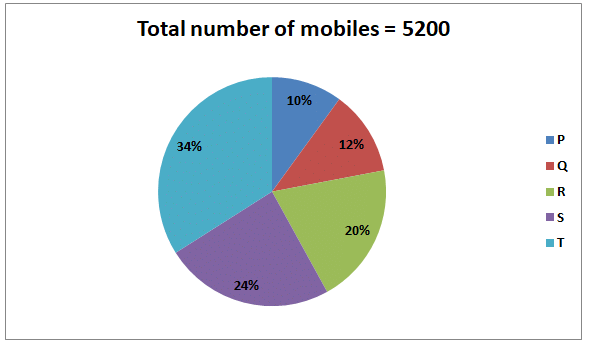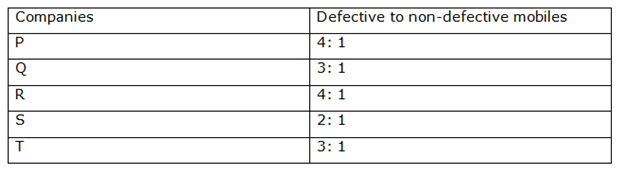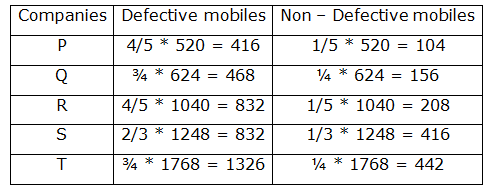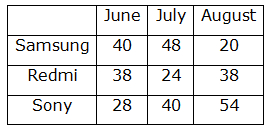Test: Quantitative Aptitude - 4

# Test: Quantitative Aptitude - 4 - Banking Exams

Test Description

## 20 Questions MCQ Test IBPS PO Mock Test Series & Past Year Papers - Test: Quantitative Aptitude - 4

Test: Quantitative Aptitude - 4 for Banking Exams 2023 is part of IBPS PO Mock Test Series & Past Year Papers preparation. The Test: Quantitative Aptitude - 4 questions and answers have been prepared according to the Banking Exams exam syllabus.The Test: Quantitative Aptitude - 4 MCQs are made for Banking Exams 2023 Exam. Find important definitions, questions, notes, meanings, examples, exercises, MCQs and online tests for Test: Quantitative Aptitude - 4 below.
Solutions of Test: Quantitative Aptitude - 4 questions in English are available as part of our IBPS PO Mock Test Series & Past Year Papers for Banking Exams & Test: Quantitative Aptitude - 4 solutions in Hindi for IBPS PO Mock Test Series & Past Year Papers course. Download more important topics, notes, lectures and mock test series for Banking Exams Exam by signing up for free. Attempt Test: Quantitative Aptitude - 4 | 20 questions in 10 minutes | Mock test for Banking Exams preparation | Free important questions MCQ to study IBPS PO Mock Test Series & Past Year Papers for Banking Exams Exam | Download free PDF with solutions
 1 Crore+ students have signed up on EduRev. Have you?
Test: Quantitative Aptitude - 4 - Question 1

### Directions: Study the following information carefully and answer the questions given below. The below chart shows the percentage distribution of mobiles manufactured in five different companies.The table shows the ratio of the defective to non-defective mobiles of five different companies.Q. Find the average number of mobiles manufactured in all the companies together.

Detailed Solution for Test: Quantitative Aptitude - 4 - Question 1

Total number of mobiles = 5200

Mobiles manufactured in P = 10% of 5200 = 520

Mobiles manufactured in Q = 12% of 5200 = 624

Mobiles manufactured in R = 20% of 5200 = 1040

Mobiles manufactured in S = 24% of 5200 = 1248

Mobiles manufactured in T = 34% of 5200 = 1768Average number of mobiles manufactured in all the companies together = 5200/5

= 1040

Test: Quantitative Aptitude - 4 - Question 2

### Directions: Study the following information carefully and answer the questions given below. The below chart shows the percentage distribution of mobiles manufactured in five different companies.The table shows the ratio of the defective to non-defective mobiles of five different companies.Q. Find the ratio of the number of non-defective mobiles of companies S and T together to the number of non-defective mobiles of companies P and Q together.

Detailed Solution for Test: Quantitative Aptitude - 4 - Question 2

Total number of mobiles = 5200

Mobiles manufactured in P = 10% of 5200 = 520

Mobiles manufactured in Q = 12% of 5200 = 624

Mobiles manufactured in R = 20% of 5200 = 1040

Mobiles manufactured in S = 24% of 5200 = 1248

Mobiles manufactured in T = 34% of 5200 = 1768Non-defective mobiles of companies S and T together = 416 + 442 = 858

Non-defective mobiles of companies P and Q together = 104+ 156 = 260

Required ratio = 429: 130

Test: Quantitative Aptitude - 4 - Question 3

### Directions: Study the following information carefully and answer the questions given below. The below chart shows the percentage distribution of mobiles manufactured in five different companies.The table shows the ratio of the defective to non-defective mobiles of five different companies.IF the ratio of the smart phones to basic phones manufactured in P and Q is 3: 1 and 2: 1 respectively, then find the difference between the number of smart phones manufactured in company P and the number of smart phones manufactured in company Q?

Detailed Solution for Test: Quantitative Aptitude - 4 - Question 3

Total number of mobiles = 5200

Mobiles manufactured in P = 10% of 5200 = 520

Mobiles manufactured in Q = 12% of 5200 = 624

Mobiles manufactured in R = 20% of 5200 = 1040

Mobiles manufactured in S = 24% of 5200 = 1248

Mobiles manufactured in T = 34% of 5200 = 1768Smart phones manufactured in company P = 3/4 * 520 = 390

Smart phones manufactured in company Q = 2/3 * 624 = 416

Required difference = 416 – 390 = 26

Test: Quantitative Aptitude - 4 - Question 4

Directions: Study the following information carefully and answer the questions given below.

The below chart shows the percentage distribution of mobiles manufactured in five different companies.The table shows the ratio of the defective to non-defective mobiles of five different companies.Q. What is the difference between the number of defective mobiles of company Q and R?

Detailed Solution for Test: Quantitative Aptitude - 4 - Question 4

Total number of mobiles = 5200

Mobiles manufactured in P = 10% of 5200 = 520

Mobiles manufactured in Q = 12% of 5200 = 624

Mobiles manufactured in R = 20% of 5200 = 1040

Mobiles manufactured in S = 24% of 5200 = 1248

Mobiles manufactured in T = 34% of 5200 = 1768Number of defective mobiles of company Q = 468

Number of defective mobiles of company R = 832

Required difference = 832 – 468= 364

Test: Quantitative Aptitude - 4 - Question 5

Directions: Study the following information carefully and answer the questions given below.

The below chart shows the percentage distribution of mobiles manufactured in five different companies.The table shows the ratio of the defective to non-defective mobiles of five different companies.Q. The number of defective mobiles of company P is approximately what percent of the total number of mobiles manufactured in all the companies together?

Detailed Solution for Test: Quantitative Aptitude - 4 - Question 5

Total number of mobiles = 5200

Mobiles manufactured in P = 10% of 5200 = 520

Mobiles manufactured in Q = 12% of 5200 = 624

Mobiles manufactured in R = 20% of 5200 = 1040

Mobiles manufactured in S = 24% of 5200 = 1248

Mobiles manufactured in T = 34% of 5200 = 1768Defective mobiles of company P = 416

Total mobiles = 5200

Required % = 416/5200 * 100

= 8%

Test: Quantitative Aptitude - 4 - Question 6

Directions: Study the following information carefully and answer the questions given below.

Q. What is the ratio of the number of Redmi headsets sold in June and July together to the number of Sony headsets sold in August month?

Detailed Solution for Test: Quantitative Aptitude - 4 - Question 6

Number of Sony headsets sold in August = 54

Redmi headsets sold in July: Sony headsets sold in August month = 4: 9

Redmi headsets sold in July = 4/9 x 54 = 24

Redmi headsets sold in July month: Sony headsets sold in June = 6: 7

Sony headsets sold in June = 7/6 x 24 = 28

Total number of Sony headsets in the three months together = 122

⇒ 28 + x + 54 = 122

⇒ x = 40 = Sony headsets sold in July

Total number of headsets sold in July month= 112

⇒ y + 24 + 40 = 112

⇒ y = 48 = Samsung headsets sold in July

Samsung headsets sold in June = Samsung headsets sold in July month – 8

= 48 – 8 = 40

Samsung headsets sold in the three months together = 108

⇒ 40 + 48 + z = 108

⇒ z = 20 = Samsung headsets sold in August

Redmi headsets sold in June = Redmi headsets sold in Aug = 40 – 2 = 38Redmi headsets sold in June and July together = 38 + 24 = 62

Sony headsets sold in August month = 54

Required ratio = 62: 54 = 31: 27

Test: Quantitative Aptitude - 4 - Question 7

Directions: Study the following information carefully and answer the questions given below.

Q. The number of Sony headsets sold by the shopkeeper in the July month is what percent of the number of Samsung headsets sold by him in the August month?

Detailed Solution for Test: Quantitative Aptitude - 4 - Question 7

Number of Sony headsets sold in August = 54

Redmi headsets sold in July: Sony headsets sold in August month = 4: 9

Redmi headsets sold in July = 4/9 x 54 = 24

Redmi headsets sold in July month: Sony headsets sold in June = 6: 7

Sony headsets sold in June = 7/6 x 24 = 28

Total number of Sony headsets in the three months together = 122

⇒ 28 + x + 54 = 122

⇒ x = 40 = Sony headsets sold in July

Total number of headsets sold in July month= 112

⇒ y + 24 + 40 = 112

⇒ y = 48 = Samsung headsets sold in July

Samsung headsets sold in June = Samsung headsets sold in July month – 8

= 48 – 8 = 40

Samsung headsets sold in the three months together = 108

⇒ 40 + 48 + z = 108

⇒ z = 20 = Samsung headsets sold in August

Redmi headsets sold in June = Redmi headsets sold in Aug = 40 – 2 = 38Sony headsets sold by the shopkeeper in the July month = 40

Samsung headsets sold by him in the August month = 20

Required % = 40/20 x 100 = 200%

Test: Quantitative Aptitude - 4 - Question 8

Directions: Study the following information carefully and answer the questions given below.

Q. Number of Samsung headsets sold in June month and July month together is approximately how much percent more/less than the number of Sony headsets sold in July and August month together?

Detailed Solution for Test: Quantitative Aptitude - 4 - Question 8

Number of Sony headsets sold in August = 54

Redmi headsets sold in July: Sony headsets sold in August month = 4: 9

Redmi headsets sold in July = 4/9 x 54 = 24

Redmi headsets sold in July month: Sony headsets sold in June = 6: 7

Sony headsets sold in June = 7/6 x 24 = 28

Total number of Sony headsets in the three months together = 122

⇒ 28 + x + 54 = 122

⇒ x = 40 = Sony headsets sold in July

Total number of headsets sold in July month= 112

⇒ y + 24 + 40 = 112

=> y = 48 = Samsung headsets sold in July

Samsung headsets sold in June = Samsung headsets sold in July month – 8

= 48 – 8 = 40

Samsung headsets sold in the three months together = 108

⇒ 40 + 48 + z = 108

⇒ z = 20 = Samsung headsets sold in August

Redmi headsets sold in June = Redmi headsets sold in Aug = 40 – 2 = 38Number of Samsung headsets sold in June month and July month together

= 40 + 48 = 88

Number of Sony headsets sold in July and August month together = 40 + 54 = 94

Required % = (94 – 88)/94 x 100 = 600/94 = 6.38

Test: Quantitative Aptitude - 4 - Question 9

Directions: Study the following information carefully and answer the questions given below.

Q. Find the difference between the number of headsets sold in June month to the number of headsets sold in August month.

Detailed Solution for Test: Quantitative Aptitude - 4 - Question 9

Number of Sony headsets sold in August = 54

Redmi headsets sold in July: Sony headsets sold in August month = 4: 9

Redmi headsets sold in July = 4/9 * 54 = 24

Redmi headsets sold in July month: Sony headsets sold in June = 6: 7

Sony headsets sold in June = 7/6 x 24 = 28

Total number of Sony headsets in the three months together = 122

⇒ 28 + x + 54 = 122

⇒ x = 40 = Sony headsets sold in July

Total number of headsets sold in July month= 112

⇒ y + 24 + 40 = 112

⇒ y = 48 = Samsung headsets sold in July

Samsung headsets sold in June = Samsung headsets sold in July month – 8

= 48 – 8 = 40

Samsung headsets sold in the three months together = 108

⇒ 40 + 48 + z = 108

⇒ z = 20 = Samsung headsets sold in August

Redmi headsets sold in June = Redmi headsets sold in Aug = 40 – 2 = 38Number of headsets sold in June month = 40 + 38 + 28 = 106

Number of headsets sold in August month = 20 + 38 + 54 = 112

Required difference = 112 – 106 = 6

Test: Quantitative Aptitude - 4 - Question 10

Directions: Study the following information carefully and answer the questions given below.

Q. If the price of a Redmi headset is 380 and the price of a Samsung headset is 420, then find the amount earned by the shopkeeper by selling the Redmi and Samsung headset in August month.

Detailed Solution for Test: Quantitative Aptitude - 4 - Question 10

Number of Sony headsets sold in August = 54

Redmi headsets sold in July: Sony headsets sold in August month = 4: 9

Redmi headsets sold in July = 4/9 x 54 = 24

Redmi headsets sold in July month: Sony headsets sold in June = 6: 7

Sony headsets sold in June = 7/6 x 24 = 28

Total number of Sony headsets in the three months together = 122

⇒ 28 + x + 54 = 122

⇒ x = 40 = Sony headsets sold in July

Total number of headsets sold in July month= 112

⇒ y + 24 + 40 = 112

⇒ y = 48 = Samsung headsets sold in July

Samsung headsets sold in June = Samsung headsets sold in July month – 8

= 48 – 8 = 40

Samsung headsets sold in the three months together = 108

⇒ 40 + 48 + z = 108

⇒  z = 20 = Samsung headsets sold in August

Redmi headsets sold in June = Redmi headsets sold in Aug = 40 – 2 = 38Redmi headsets sold in August = 38

Samsung headsets sold in August = 20

Amount earned by the shopkeeper by selling the Redmi and Samsung headset in August month = 38 x 380 + 20 x 420 = Rs.22840

Test: Quantitative Aptitude - 4 - Question 11

A box contains 36 balls of three different colors white, purple and violet and the number of purple balls is 25% less than that of the number of white balls in the box. If the probability of drawing a violet ball is 5/12, then find the probability of drawing exactly two purple balls?

Detailed Solution for Test: Quantitative Aptitude - 4 - Question 11

Total number of balls = 36

The number of violets balls = x

xC1/36C= 5/12

x/36 = 5/12

x = 15

The number of white and purple balls=36 - 15 = 21

The number of purple balls  21 x 3/7 = 9

Required probability = 9C2/36C2

=2/35

Test: Quantitative Aptitude - 4 - Question 12

A and B started the business with the investment of Rs.1440 and Rs.3600 respectively. After 4 months, A added Rs.1760 and C joined them with the investment of Rs.3240. At the end of the year, the profit share of C is what percentage of the profit share of B?

Detailed Solution for Test: Quantitative Aptitude - 4 - Question 12

The profit share of A, B and C
= (1440 x 4 + 3200 x 8): 3600 x 12: 3240 x 8

= 784: 1080: 648

= 98: 135: 81

Required percentage=81/135 x 100 = 60%

Test: Quantitative Aptitude - 4 - Question 13

The marked price of the toaster is Rs.300 more than its cost price and the shopkeeper offers a discount of 20% on the marked price of the toaster. If he gets a profit of 12%, then find the profit earned by the shopkeeper?

Detailed Solution for Test: Quantitative Aptitude - 4 - Question 13

CP = x

MP = x + 300

(x+300) x 80/100 - x = x X 12/100

4x/5 + 240 - x = 3x/25

4x + 1200 - 5x = 3x/5

20x + 6000 - 25x = 3x

8x=6000

x=750

Profit=750*12/100=Rs.90

Test: Quantitative Aptitude - 4 - Question 14

Vessel A contains 62.5% of milk and the remaining 18 liters are water mixed with another vessel B contains 75 liters of a mixture of milk and water in that of 2:1 respectively. If x liters of water are added to it, then the ratio of milk and water becomes 8:5, then find the value of x?

Detailed Solution for Test: Quantitative Aptitude - 4 - Question 14

Milk in vessel A = 18 x 5/3 = 30 liters

Water in vessel A = 18 liters

Milk in vessel B = 75 x 2/3 = 50 liters

Water in vessel B = 75 - 50 = 25 liters

(30 + 50)/(18 + 25 + x) = 8/5

80/(43 + x) = 8/5

400 = 344 + 8x

x = 7 liters

Test: Quantitative Aptitude - 4 - Question 15

The speed of the stream is 33.33% of the speed of the boat. If the speed of the stream is decreased by 5 kmph, then the downstream speed of the boat is 75 kmph, then find the time taken by the boat to cover 360 km upstream after decreasing the speed of the stream?

Detailed Solution for Test: Quantitative Aptitude - 4 - Question 15

Speed of the boat = 3x

Speed of the stream = 3x * 1/3 = 1x

3x + (x - 5) = 75

4x = 80

x = 20

Speed of the boat = 3 x 20 = 60 kmph

Speed of the stream is decreased by 5 kmph = 20 - 5=15 kmph

Upstream speed of the boat
= 60 - 15 = 45 kmph

Required time=360/45=8 hours

Test: Quantitative Aptitude - 4 - Question 16

Directions: Following questions contain two equations as I and II. You have to solve both the equations and determine the relationship between them and give an answer as,

I) x2 – 3x – 40 = 0

II) y2 + 8y – 240 = 0

Detailed Solution for Test: Quantitative Aptitude - 4 - Question 16

x2 – 3x – 40 = 0

x2 – 8x + 5x – 40 = 0

x(x – 8) + 5(X – 8) = 0

x = 8, -5

y2 + 8y – 240 = 0

y2 + 20y – 12y – 240 = 0

y(y + 20) – 12(y + 20) = 0

y = 12, -20

Relationship between x and y cannot be established.

Test: Quantitative Aptitude - 4 - Question 17

What approximate value should come in the place of (?) in the following questions.

839.39 - ?=√960 + 16.32 x 24.88

Detailed Solution for Test: Quantitative Aptitude - 4 - Question 17

839.39 - ? = √960 + 16.32 x 24.88

839 - ? = 31 + 400

? = 408

Test: Quantitative Aptitude - 4 - Question 18

What approximate value should come in the place of (?) in the following questions.

8?- 14.78 x 19.99 = 29.78 x 6.8/5.2 + 170.24

Detailed Solution for Test: Quantitative Aptitude - 4 - Question 18

8- 14.78 x 19.99 = 29.78 x 6.8/5.2 + 170.24

8? = 42 + 170 + 300

8= 512

? = 3

Test: Quantitative Aptitude - 4 - Question 19

Directions: Following questions contain two equations as I and II. You have to solve both the equations and determine the relationship between them and give an answer as,

I) (x – 4)2 = 81

II) y2 + 17y = - 60

Detailed Solution for Test: Quantitative Aptitude - 4 - Question 19

(x – 4)2 = 81

x2 + 16 – 8x = 81

x2 – 8x – 65 = 0

x2 – 13x + 5x – 65 = 0

x(x – 13) + 5(x – 13) = 0

x = 13, -5

y2 + 17y = - 60

y2 + 12y + 5y + 60 = 0

y(y + 12) + 5(y + 12) = 0

y = -12, -5

x ≥ y

Test: Quantitative Aptitude - 4 - Question 20

What approximate value should come in the place of (?) in the following questions.

45.22% of 959.99-? = 84.32 x 24.88/21.41

Detailed Solution for Test: Quantitative Aptitude - 4 - Question 20

45.22% of 959.99-? = 84.32 x 24.88/21.41

45 x 960/100 - 84 x 25/21 = ?

432 – 100 = ?

? = 332

## IBPS PO Mock Test Series & Past Year Papers

33 docs|72 tests
Information about Test: Quantitative Aptitude - 4 Page
In this test you can find the Exam questions for Test: Quantitative Aptitude - 4 solved & explained in the simplest way possible. Besides giving Questions and answers for Test: Quantitative Aptitude - 4, EduRev gives you an ample number of Online tests for practice

33 docs|72 tests